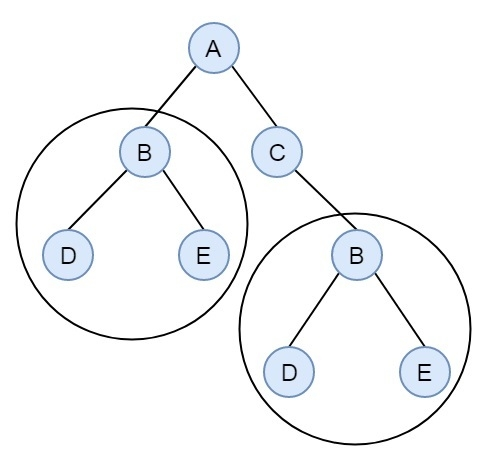# Check if a Binary Tree contains duplicate subtrees of size 2 or more in C++

Consider we have a binary tree. We have to find if there are some duplicate subtrees of size 2 or more in the tree or not. Suppose we have a binary tree like below −There are two identical subtrees of size 2. We can solve this problem by using tree serialization and hashing process. The idea is serializing the subtrees as string, and store them in hash table. Once we find a serialized tree which is not leaf, already exists in hash table, then return true.

## Example

Live Demo

#include <iostream>
#include <unordered_set>
using namespace std;
const char MARKER = '$'; struct Node { public: char key; Node *left, *right; }; Node* getNode(char key) { Node* newNode = new Node; newNode->key = key; newNode->left = newNode->right = NULL; return newNode; } unordered_set<string> subtrees; string duplicateSubtreeFind(Node *root) { string res = ""; if (root == NULL) // If the current node is NULL, return$
return res + MARKER;
string l_Str = duplicateSubtreeFind(root->left);
if (l_Str.compare(res) == 0)
return res;
string r_Str = duplicateSubtreeFind(root->right);
if (r_Str.compare(res) == 0)
return res;
res = res + root->key + l_Str + r_Str;
if (res.length() > 3 && subtrees.find(res) != subtrees.end()) //if subtree is present, then return blank string return "";
subtrees.insert(res);
return res;
}
int main() {
Node *root = getNode('A');
root->left = getNode('B');
root->right = getNode('C');
root->left->left = getNode('D');
root->left->right = getNode('E');
root->right->right = getNode('B');
root->right->right->right = getNode('E');
root->right->right->left= getNode('D');
string str = duplicateSubtreeFind(root);
if(str.compare("") == 0)
cout << "It has dublicate subtrees of size more than 1";
else
cout << "It has no dublicate subtrees of size more than 1" ;
}

## Output

It has dublicate subtrees of size more than 1